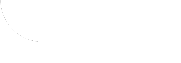Home Normal Version IE Version Search | BBS | Guest Book | Site Map | Help | Contact us | About us
 Light version
Introduction
Essence
Memorizing

Basic Formulas
Sum and Difference Formulas
Double & Triple Angle Formulas
Half Angle Formulas
Product to Sum Formulas
Sum to Product Formulas
All Formulas

Understanding
Summarizing
Examples
Exercises
Final thoughts

## 3. How to Memorize the Given Identities?

Here, we will recommend ways to memorize the basic trigonometric identities. It is important to memorize these identities in order to aid you in solving trigonometric problems. If you already know these formulas, that is great, if you don't know, this is only one suggestion to memorize these identities. If you have your own way of learning these then use it; looking to this page for some tips.

There are five major sections to the formulas: Sum and Difference formulas, Double-Angle formulas, Half-Angle formulas, Product-to-Sum formulas, and Sum-to-Product formulas. There are some basic formulas you should already know. Many of these are related to each other and it is easier to memorize them if you know some basic formulas. Also, notice the groupings of the different formulas inside each section, if one is memorized then another may be just a simple modification of it.LWR